# Linear Regression: Theory and Code from Scratch

## Introduction

Have you ever thought about how the forecast for tomorrow or the coming month is predicted? How do professionals expect the company’s profits or losses in the next five years? Or how the medical practitioners predict when will be the peak wave of the covid-19 virus. It is all done with the help of regression, which belongs to the family of statistics. Regression analysis is a method to know how a dependent(target) variable changes concerning an independent(predictor) variable. Regression is of various types, but linear regression is one of the most uncomplicated and demanding Machine Learning algorithms.

## What is Linear regression?

Linear Regression is a category of Supervised machine Learning which shows a linear relationship between a dependent variable(y) and one or more independent variables(x); hence it justifies the name linear regression.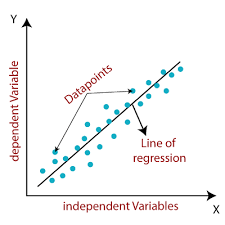Mathematically, it can be represented as

Y = mx + b

Where,

Y- dependent variable

X- independent variable

b- Bias coefficient / error

M- is the slope

## Types of Linear Regression

There are two types of Linear regression:

• Simple Linear Regression: It is the type of regression where the numeric value of a dependent variable is predicted using only one independent variable. For example, a person’s salary can be calculated by the number of years experience s(he) has.
• Multiple Linear Regression: This type of regression where the numeric value of a dependent variable is predicted using more than one independent variable.

## Linear Regression Line

There are two types of regression lines:

• Positive Linear Regression: If the dependent variable increases with the increase in the independent variable, then it is a positive linear regression.
• Negative Linear Regression- If the dependent variable decreases with the increase in the independent variable, then it is a negative linear regression. Here the equation for the regression line will be: y = -mx + b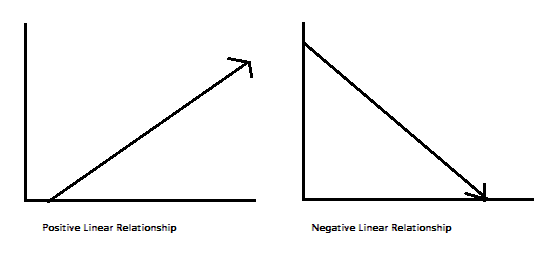## Finding the best-fit Regression line

While working on a Linear Regression model, our primary goal is to find the best-fit regression line; the inaccuracy or difference between the actual value and predicted value should be minimal. The best-fit line of regression will have the least miscalculations.

## Assumptions of Linear Regression

The assumptions of the linear regression model are as follows:

• Linearity: Linear regression states that the dependent and independent variables should be linearly related to check that we can plot a scatter plot.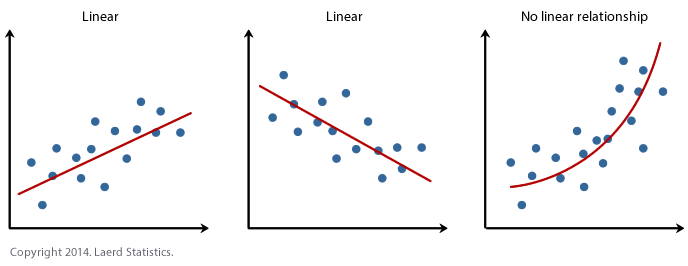Source: Link

• Normality: The dependent and independent variables should be normally distributed, which means that the majority of the values must be around the mean, although there could be some outlier exceptions.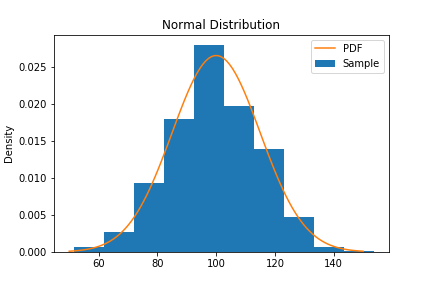• Independence/ Multicollinearity: There should be no collinearity between the independent variables. To check that, we can plot a heatmap/ correlation matrix.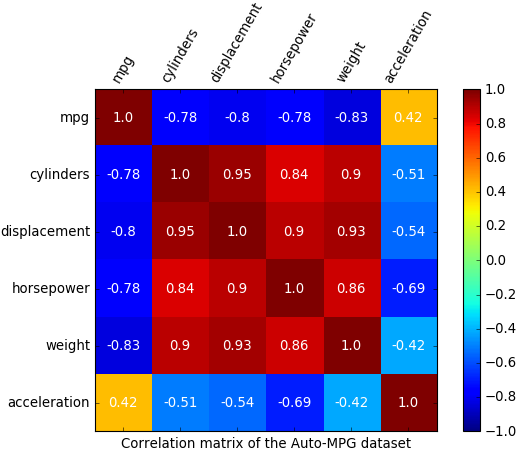## How to deal with breaches in assumptions?

The violation in any of the assumptions leads to inaccuracy of the model and predicts wrong outputs.

To overcome a situation like this, there are various learning model types.

• Simple Linear Regression: When there is a single input, we can calculate the statistical properties of the data, such as mean, standard deviation, covariance, and correlation. The equation for simple linear regression is: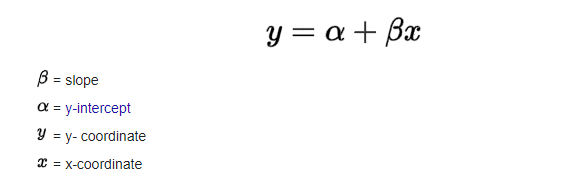• Ordinary Least Square: When there is more than one input variable, we can use ordinary least squares to estimate the values of the coefficient. In this procedure, we calculate the distance between the data points and the line of regression, square it and add up all the errors together(This value is known as Mean Squared Error). This value is reduced in Ordinary Least Square. The mathematical representation of ordinary least square is: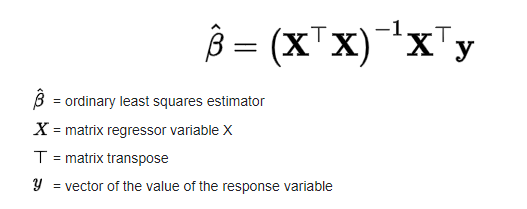• Gradient Descent: When there is more than one input variable, we use a process called Gradient Descent in which we iteratively minimize the errors. The process works by starting with random values for each coefficient. For each set of input and output values, the sum of squared errors is calculated. A learning rate is used as a scale factor; the coefficients are updated by minimizing the mistakes. The process is replicated until a minimum sum squared error is achieved or no further improvement is possible.
• Regularization: In this method, we are supposed to minimize the squared error of the model on the training data and reduce the complexity of the model.
Two famous examples of regularization procedures for linear regression are:
Lasso Regression: where Ordinary Least Squares are modified to minimize the absolute sum of the coefficients (called L1 regularization). Mathematically it can be represented as: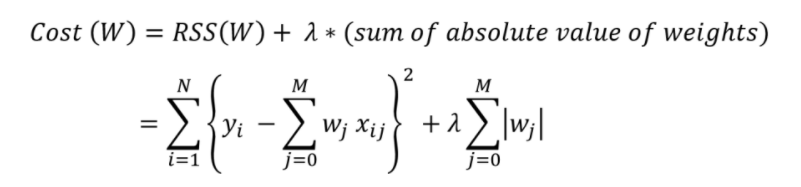Where

RSS is the residual sum of squares

Yi is the dependent variable

Xij is the dependent variable

Wj is the differentiation of cost with respect to particular data points.

Ridge Regression: where Ordinary Least Squares are modified to minimize the squared absolute sum of the coefficients (called L2 regularization). Mathematically it can be represented as: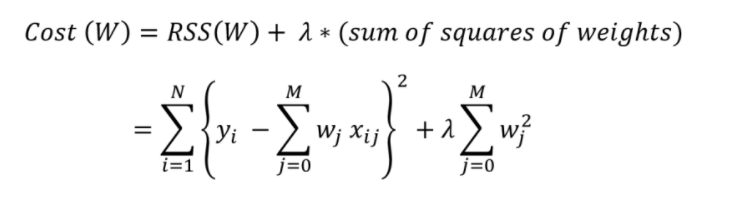Where,

RSS is the residual sum of squares

Y is the dependent variable

X is the independent variable

## Linear Regression: Code

We will work on the salary dataset to predict an individual’s salary based on given years of experience.

First, we’ll import all the necessary libraries and the dataset.

``````#import necessary libraries
import pandas as pd
import numpy as np
import math
import operator
import matplotlib.pyplot as plt
%matplotlib inline``````

Next, we’ll load our dataset in CSV form as a pandas dataframe and assign the values of x and y. In this case, the salary is dependent on years of experience; therefore, x will be years of experience, and y will be the salary.

``````# uploading data file from local drive in google colab and reading csv file as pandas dataframe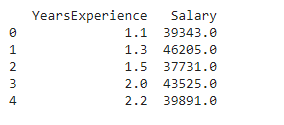These are the first five rows of our dataset.

Now we will write the class for linear regression function:

``````class linear_regression_code:
# assigning the values of x & y
X = data['YearsExperience'].values
Y = data['Salary'].values
plt.figure(figsize = (10,5))
plt.scatter(X,Y)
def linear_regression(x, y):
#len() is an inbuilt function used to calculate the length of variable.
N = len(x)
x_mean = x.mean()
y_mean = y.mean()
#Now we will calculate B1 which is slope and B0 which is the intercept.
B1_num = ((x - x_mean) * (y - y_mean)).sum()
B1_den = ((x - x_mean)**2).sum()
B1 = B1_num / B1_den
B0 = y_mean - (B1*x_mean)
reg_line = 'y = {} + {}β'.format(B0, round(B1, 3))
return (B0, B1, reg_line)
N = len(X)
x_mean = X.mean()
y_mean = Y.mean()
#Calculating numerator and deniminator seperately
B1_num = ((X - x_mean) * (Y - y_mean)).sum()
B1_den = ((X - x_mean)**2).sum()
B1 = B1_num / B1_den
B0 = y_mean - (B1 * x_mean)
def corr_coef(x, y):
N = len(x)
num = (N * (x*y).sum()) - (x.sum() * y.sum())
den = np.sqrt((N * (x**2).sum() - x.sum()**2) * (N * (y**2).sum() - y.sum()**2))
R = num / den
return R
B0, B1, reg_line = linear_regression(X, Y)
print('Regression Line: ', reg_line)
R = corr_coef(X, Y)
print('Correlation Coef.: ', R)
print('R square value: ', R**2)
def predict(B0, B1, new_x):
y = B0 + B1 * new_x
return y
plt.figure(figsize=(12,5))
plt.scatter(X, Y, s=300, linewidths=1, edgecolor='black')
text = '''X Mean: {} Years
Y Mean: \${}
R: {}
R^2: {}
y = {} + {}X'''.format(round(X.mean(), 2),round(Y.mean(), 2),round(R, 4),round(R**2, 4),round(B0, 3),round(B1, 3))
plt.text(x=1, y=100000, s=text, fontsize=12, bbox={'facecolor': 'grey', 'alpha': 0.2, 'pad': 10})
plt.title('How Experience Affects Salary')
plt.xlabel('Years of Experience', fontsize=15)
plt.ylabel('Salary', fontsize=15)
plt.plot(X,B0 + B1*X,c = 'r',linewidth=5,alpha=.5,solid_capstyle='round')
plt.scatter(x=X.mean(), y=Y.mean(), marker='*', s=10**2.5, c='r')#average point
reg_line = 'y = {} + {}β'.format(B0, round(B1, 3))``````

In the code, we have calculated the slope of B1.

After calculating B1, we have found the value of B0 from the formula.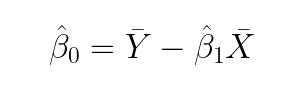Where

B0: is the intercept

Ý: is Ymean

B1: is the slope

``B0 = y_mean - (B1 * x_mean)``

To determine if it is best-fit and to check the efficiency of our regression line, we have calculated the correlation coefficient, also known as R. and R², respectively. For that, we have defined the function corr_coef().

This code will give the following output.

``````Regression Line:  y = 25792.20019866869 + 9449.962β
Correlation Coef.:  0.97824161848876
R square value:  0.9569566641435087``````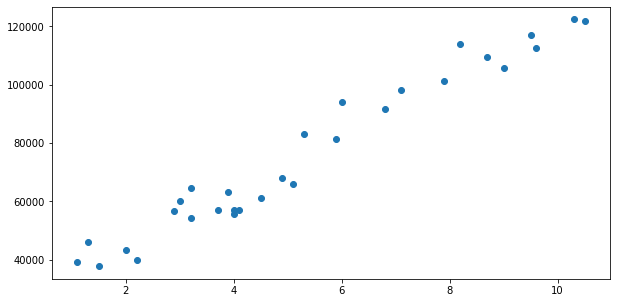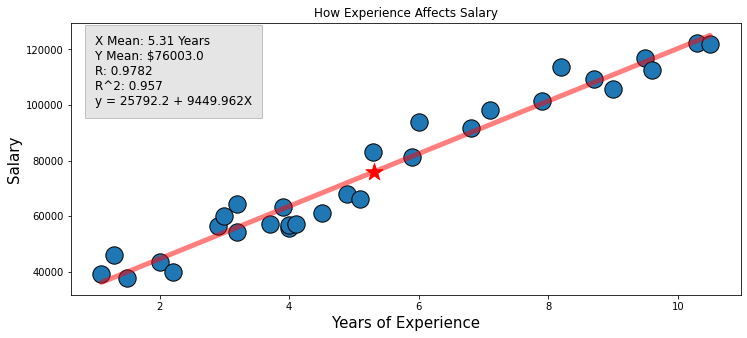1. What are the most critical assumptions of Linear Regression?
Ans: There are three critical assumptions. First, there has to be a linear relationship between the independent and dependent variables. Secondly, there must be no or significantly less multicollinearity between the independent variables in the dataset. The value needs to be confined, which depends on the domain requirement. The third is homoscedasticity. It is one of the essential assumptions which states that the errors are equally distributed.

2. What is heteroscedasticity?
Ans: Heteroscedasticity is the opposite of homoscedasticity, which means that the errors are unequally distributed.

3. What are some advantages of linear regression?
Ans: Linear regression has a considerably lower time complexity when compared to some of the other machine learning algorithms. The mathematical equations of Linear regression are also reasonably easy to understand and interpret. Hence Linear regression is effortless to master.

## Key Takeaways

In this article, we have studied linear regression, various types of linear regression, line of regression, finding the best-fit line of regression, assumptions of linear regression, taking care of these assumptions, and practical implementation of Linear Regression-looking to build a career in Data Science? Check out our industry-oriented machine learning course curated by our faculty from Stanford University and Industry experts.